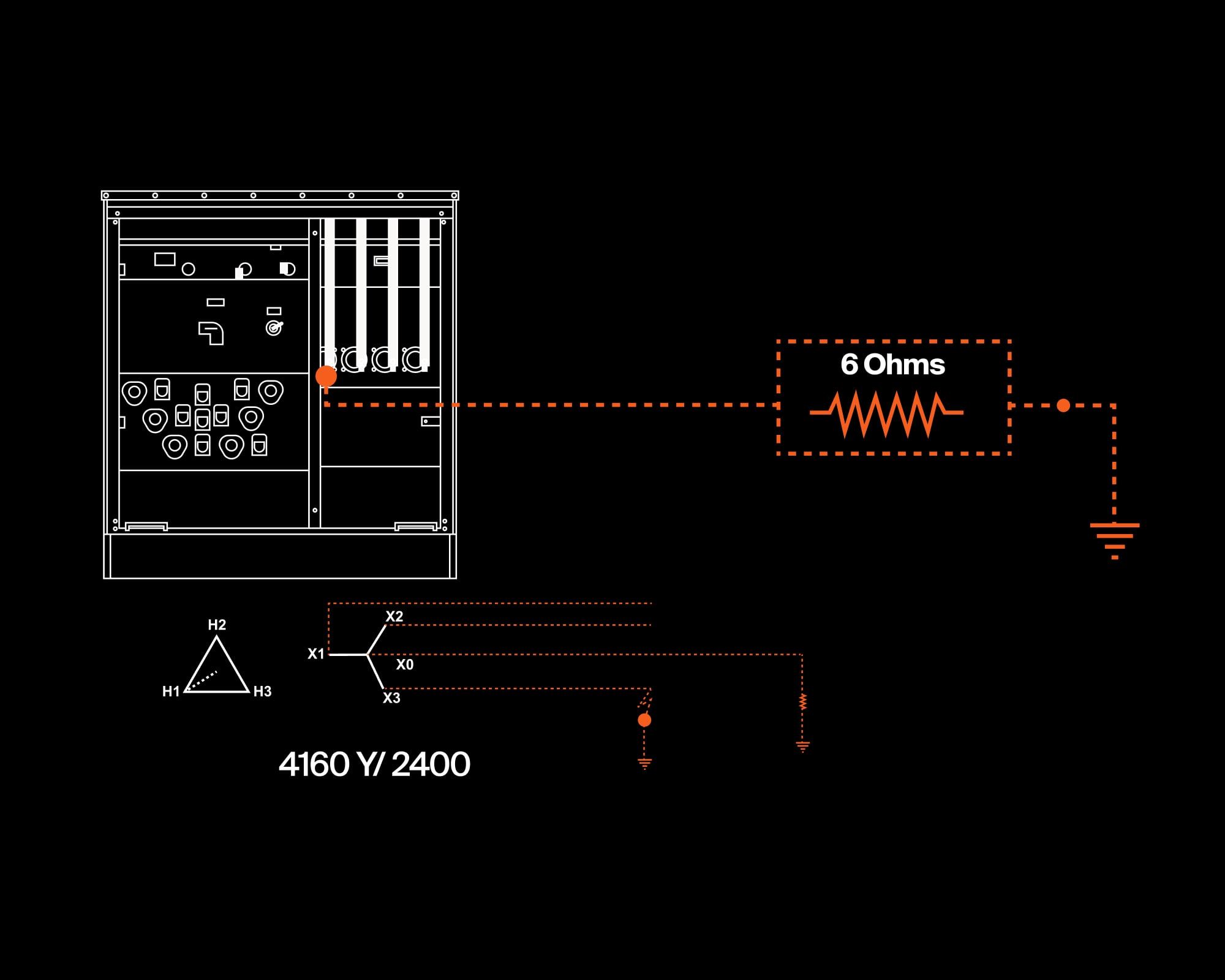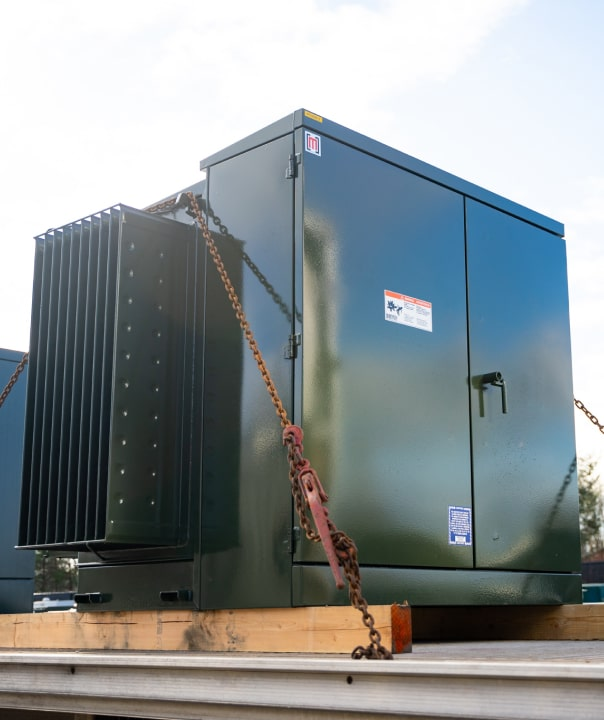# Ohm’s & Joule’s Law: Transformer & Electrical Formulas

Learn to use Ohm's and Joule's Law to size transformers and calculate power consumption.

November 2, 2023## What is current, voltage, and resistance?

When dealing with transformers and electricity in general, there are three main elements in play–resistance, voltage, and current. We’ll briefly define each of these elements and then look at a couple of formulas that help break down these relationships in transformers and electrical circuits.

### Current

Current is the flow of electrons through a conductor in a circuit.

### Voltage

Voltage is the pressure that pushes the current through the conductor in a circuit.

### Resistance

Resistance (measured in ohms) is the force that opposes the flow of current through a circuit.

The figure below represents the relationship between these three elements.

## Resistance Formulas

In any kind of electrical work, it’s hard to go far without encountering Ohm’s Law. Ohm’s Law, named after physicist Georg Ohm, is a fundamental concept in electrical engineering for understanding circuits with resistive elements.

### What is Ohm’s Law?

Ohm’s Law expresses the fundamental relationship between voltage, current, and resistance in a circuit. Ohm’s Law states that:

Where the resistance in a conductor is constant, the current passing between two points in that conductor is directly proportional to the voltage across the two points.

### Ohm’s Law Equation

This relationship is expressed by the following equation:

V = IR

Where…

V = Voltage (measured in Volts)

I = Current (measured in Amps)

R = Resistance (measured in Ohms)

Traditionally the letter “E” is used to represent voltage, but “V” is also commonly used now. For the purpose of this article, we will use the letter “V” to represent voltage.

We can also rearrange the equation to solve for resistance, current, or voltage respectively as shown in the figure below.

Now, let’s put one or more of the above forms of Ohm’s Law into practice with a real-life situation with a constant resistance value–such as a neutral grounding resistor (or NGR). Low resistance grounded systems depend on a resistor to limit the amount of fault current during a line to ground fault. This is done by connecting a resistance between the neutral point of the transformer and earth ground.

Let’s assume we needed to size a neutral ground resistor to limit fault current around 100 to 400 amps in a 4160Y/2400 system. We can use the following version of Ohm’s Law to calculate the size of the NGR…

R=V/I

To find the voltage, we will divide the line voltage by √3.

4160 / √3 = 2400V

Next, we will input the maximum allowable fault current value at 2400 volts.

R = 2400V / 400A = 6 Ohms

For this particular low resistance grounded system, an NGR with a resistor rated for 6 Ohms will be required.

To illustrate the relationship between voltage and current when resistance is constant, let’s now plug in a fault current value of 300 amps to measure the voltage–with the same 6 Ohm resistance.

Since we are solving for voltage, we will use this version of our Ohm’s Law equation…

V = IR

V = 300A * 6 Ohms = 1,800 V

When the fault current is lowered to 300 amps, the line to neutral voltage will be 1,800 volts. We can see that when resistance is constant, the voltage will decrease as the current decreases and vice versa.

The resistor in the above example is referred to as an Ohmic device since it operates within the parameters of Ohm’s Law. Any component in an electrical circuit that maintains the same resistance value regardless of the current or voltage can be referred to as Ohmic.

Ohm’s Law is a fundamental part of electrical engineering for designing and analyzing circuits with resistive elements. With inductive circuits, directly applying Ohm’s Law becomes a bit more complicated (like between a transformer’s primary and secondary windings), and this is a bit beyond the scope of this article.

## Power Formulas

Another law which is often referred to alongside Ohm’s Law, which is frequently used for power calculations is Joule’s Law.

### What is Joule’s Law?

Joule’s Law deals with calculations involving electrical power–measured in watts or (in the case of electric transformers) VA. Power calculations are a fundamental part of any electrical system, and they are key in sizing transformers. When it comes to selecting the right transformer, the first order of business is usually to make sure the size of the transformer will support the load it serves (making sure the transformer core and coils are large enough to handle the amount of current flowing through them).

### Joule’s Law Equation

P = VI

Where…

P = Power (measured in Watts or volt-amperes)

V = Voltage (measured in volts)

I = Current (measured in amperes)

## Using Ohm’s Law & Joule’s Law Together

At first glance you will notice that resistance is not shown in the above form of the equation for Joule’s Law. Instead of R (resistance), we have V (voltage) and I (current) represented. This is another form of the Joule’s Law equation which we commonly use for power calculations.

Here, we can integrate the following Ohm’s Law formula to incorporate the variable for resistance.

Ohm’s Law… V=IR

Joule’s Law… P=VI

P=IR*I

Simplified… P = I²R

Now, if we have the resistance and current values only, we can solve for power.

## Ohm’s Law Wheel

This kind of interchange between Joule’s Law and Ohm’s Law is frequently employed to solve for different variables in an electrical circuit or device. This relationship is expressed in what is referred to as the Ohm’s Law Wheel.

## Power Calculations and Transformer Sizing

The formula which is used most frequently when sizing transformers for a load is P=VI (or Watts = Voltage x Current). This is the equation which represents the transformer’s capacity (VA). For instance, if you have a single phase load that operates at 480 volts with a continuous current rating of 60 amps, you would go about sizing the transformer as follows…

Operating voltage = 480 Volts

Continuous load rating = 60 Amps

480V * 60A = 28,800 VA

28,800 / 1,000 (to convert to kVA) = 28.8 kVA

This same formula applies to three phase transformers as well. In order to account for the other phases, we will incorporate the square root of three.

√3 = 1.732

kVA = (1.732 * V * A) / 1,000

So, for a three phase load which runs at 480 volts with a continuous current rating of 100 amps, we could solve for the transformer size as follows….

kVA = (1.732 * 480 * 100) / 1,000

kVA = 83.14

## Considering Efficiency & Power Factor

Let’s go one step further and look at applications involving Joule’s Law where electrical energy is converted to (or lost as) heat energy.

Equipment, no matter how efficient, will use up a certain amount of power to operate. This operating power is not used in accomplishing the work the equipment is designed to perform, it is simply the energy required to keep things running. Motors tend to need more of this extra power to keep things going, which means you’ll need to buffer in a little extra headroom when sizing transformers.

For instance, if the total connected motor load is rated for 60 amps continuous in a circuit, the actual demand that circuit puts on the transformer could be something like 72 amps when you factor in the additional power that is wasted in keeping the motor running (60 amps to perform the actual work the motor needs to do, and 12 amps just to operate so to speak).

This additional demand should be taken into account as well when you calculate the peak load the transformer will see. If a motor has an efficiency rating of 80% (like the example above), then, an additional 20% will need to be factored into the initial load calculation to ensure the transformer is not undersized.

### What is power factor

We refer to this ratio between the power used to perform the actual work the load is designed for (measured in watts) and the total power consumed by the circuit (measured in volt amperes or VA) as Power Factor. The value for power factor will always be below 1 (unity), since there is always some power loss in an electrical system. A good power factor would be as close to 1 as possible (0.9 through 0.99). Systems that waste more energy will have a lower power factor (0.8 and lower for example).

## Conclusion

Ohm’s Law and Joule’s Law help explain the relationship between resistance, power, voltage, and current. Both help to illustrate the electrical concepts that surround transformers and serve as an indispensable part of electrical projects related to transformer sizing and application. Please fill out the form below with any questions related to these formulas and sizing your transformer.